﻿ 小水线面双体船支柱斜度对其耐波性能影响分析
 舰船科学技术2016, Vol. 38Issue (6): 20-23PDF

1. 江苏科技大学 船舶与海洋工程学院, 江苏 镇江 212003 ;
2. 江苏现代造船技术有限公司, 江苏 镇江 212003

Influence analysis of the seakeeping performance for SWATH with inclined strut
LI Dong-qin1,2, LIU Cun-jie1, XU Shi-you1
1. School of Naval Architecture and Ocean Engineering, Jiangsu University of Science and Technology, Zhenjiang 212003, China ;
2. Jiangsu Modern Shipbuilding Technology Co. Ltd., Zhenjiang 212003, China
Abstract: Based on the three-dimensional potential flow theory, this paper studied the seakeeping performance of Small Water-plane Area Twin Hull (SWATH) with different inclined struts at the design speed. Combining the motion response amplitude function in regular wave with wave spectrum, we get the significant statistics of motion response. By comparing the frequency response curves of different inclined struts under the same sea state condition, we find that as inclined angle increases, the peak value of pitch and heave reduces. It turns out that inclined strut increases the added mass and damping of ship motion, weaken the resonance peak.
Key words: SWATH     seakeeping performance     inclined strut
0 引言

1 基本理论

1.1 基本方程及边界条件

 $\frac{{{\partial ^2}\varPhi }}{{{\partial ^2}{x^2}}} + \frac{{{\partial ^2}\varPhi }}{{{\partial ^2}{y^2}}} + \frac{{{\partial ^2}\varPhi }}{{{\partial ^2}{z^2}}} = 0\text{，}$ (1)

 $\begin{array}{l} \displaystyle \frac{{{\partial ^{\rm{2}}}\varPhi }}{{\partial {{{t}}^2}}} \displaystyle + \frac{{g\partial \varPhi }}{{\partial y}} + \frac{1}{2}\nabla (\nabla \varPhi \cdot \nabla \varPhi ) + 2\nabla \varPhi \cdot \nabla \frac{{\partial \varPhi }}{{\partial t}} = 0\text{。} \end{array}$ (2)

1）自由面边界条件：

 $\begin{array}{l} -{\omega ^2}{\phi _j} \!+\! 2{U_0}i\omega \displaystyle \frac{{\partial {\phi _{\rm{j}}}}}{{\partial x}} \!+ \!{U_0}^2\frac{{\partial {\phi _{^j}}^2}}{{\partial {x^2}}} \!+\! g\frac{{\partial {\phi _j}}}{{\partial z}} \!=\! 0,{{j = 1,2 \ldots ,7}}\text{；} \end{array}$ (3)

2）物面条件：

 $\left. \begin{array}{l} \displaystyle-\frac{{\partial {\phi _j}}}{{\partial n}} = {n_j},j = 1,2...,6\\ \displaystyle \frac{{\partial {\phi _7}}}{{\partial n}} = \frac{{\partial {\phi _0}}}{{\partial n}} \end{array} \right\}\text{；}$ (4)

3）水底条件：$$\displaystyle \frac{{\partial {\phi _j}}}{{\partial n}} = 1,2,...,7\text{；}$$

4）辐射条件：无穷远处有波浪向外传播

1.2 波浪谱

 ${{S}}(\omega ) = \frac{{{H_s}^2{T_z}}}{{8{\pi ^2}}}{(\frac{{\omega {T_z}}}{{2\pi }})^{-5}} {e^{\frac{{-1}}{\pi }{{(\frac{{\omega {T_z}}}{{2\pi }})}^{-4}}}}\text{。}$ (5)

1.3 响应函数与运动响应特征值

 ${\rm{E}} = \int_0^\infty {{{(\frac{{{\xi _k}}}{A})}^2}S(\omega ){\rm{d}}\omega } \begin{array}{*{20}{c}} {} \end{array} \text{。}$ (6)

1.4 粘性阻尼

 ${\beta _{\rm{0}}} = {\rm{2}}\sqrt {(M + {M_a}) \times {C_i}} \text{。}$ (7)

2 数值计算模型及参数设置 2.1 小水线面双体船主尺度表 1 船舶主尺度 Tab.1 Main particulars of the SWATH
2.2 计算模型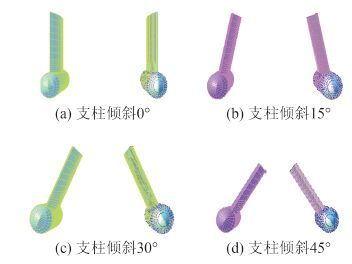图 1 不同支柱倾斜角度的小水线面双体船 Fig. 1 Different inclined angle of SWATH vessels
2.3 计算参数设置

3 计算结果对比与分析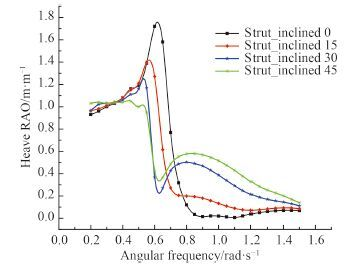图 2 迎浪垂荡响应函数（180°） Fig. 2 Heave response function in head sea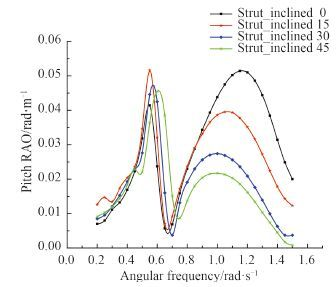图 3 迎浪纵摇响应函数（180°） Fig. 3 Pitch response function in head sea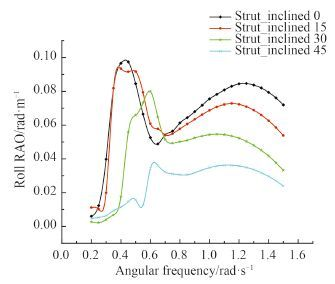图 4 横浪横摇响应函数（90°） Fig. 4 Roll response function in beam sea表 2 典型浪向角下各自由度运动有义统计值 Tab.2 Significant statistics of different degree of freedom in typical wave direction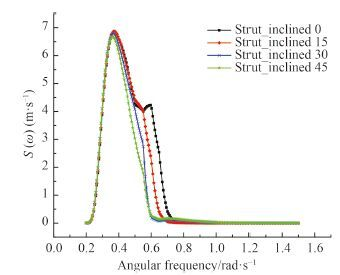图 5 迎浪垂荡响应谱（180°） Fig. 5 Heave response spectrum in head sea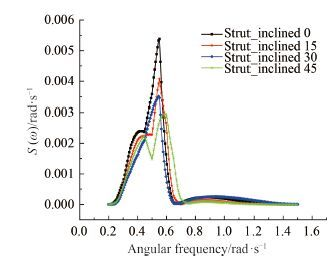图 6 迎浪纵摇响应谱（180°） Fig. 6 Pitch response spectrum in head sea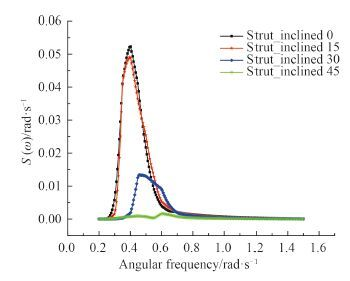图 7 横浪横摇响应谱（90°） Fig. 7 Roll response spectrum in beam sea

4 结语

  QIAN P, YI H, LI Y H. Numerical and experimental studies on hydrodynamic performance of a small-waterplane-area-twin-hull (SWATH) vehicle with inclined struts[J]. Ocean Engineering , 2015, 96 :181–191. DOI:10.1016/j.oceaneng.2014.12.039  BRIZZOLARA S, CURTIN T, BOVIO M, et al. Concept design and hydrodynamic optimization of an innovative SWATH USV by CFD methods[J]. Ocean Dynamics , 2012, 62 (2) :227–237. DOI:10.1007/s10236-011-0471-y  LATORRE R, VASCONSELLOS J. Study of hull angle influence on SWATH heave and pitch motions[J]. Naval Engineers Journal , 2001, 113 (1) :63–70. DOI:10.1111/nej.2001.113.issue-1  黄鼎良. 小水线面双体船性能原理[M]. 北京: 国防工业出版社, 1993 : 110 . HUANG Ding-liang. SWATH performance principle[M]. Beijing: National Defense Industry Press, 1993 : 110 .  Sesam HydroD User Tutorial. Global response of a damaged semi-submersible offshore platform in Frequency domain by Wasim and Wadam  RODDIER D, PEIFFER A, AUBAULT A, et al. A generic 5 MW WINDFLOAT for numerical tool validation & comparison against a generic spar[C]//Proceedings of the ASME 201130th International Conference on Ocean, Offshore and Arctic Engineering. Rotterdam:ASME, 2011:2011-50278  冯炎鑫. 三体船在波浪上的运动响应计算[D]. 天津:天津大学, 2013. FENG Yan-xin. Motion response calculation of trimaran in wave[D]. Tianjin:Tianjin University, 2013.  王涵. 小水深半潜型风电浮式基础的耦合动力分析与试验研究[D]. 天津:天津大学, 2013:26-28. WANG Han. Coupling dynamic analysis and experimental research of semi-submersible floating foundation for wind power in the shallow water[D]. Tianjin:Tianjin University, 2013:26-28.  FALTINSEN O M. 船舶与海洋工程环境载荷[M]. 杨建民, 肖龙飞, 葛春花, 译. 上海:上海交通大学出版社, 2008:24-25.